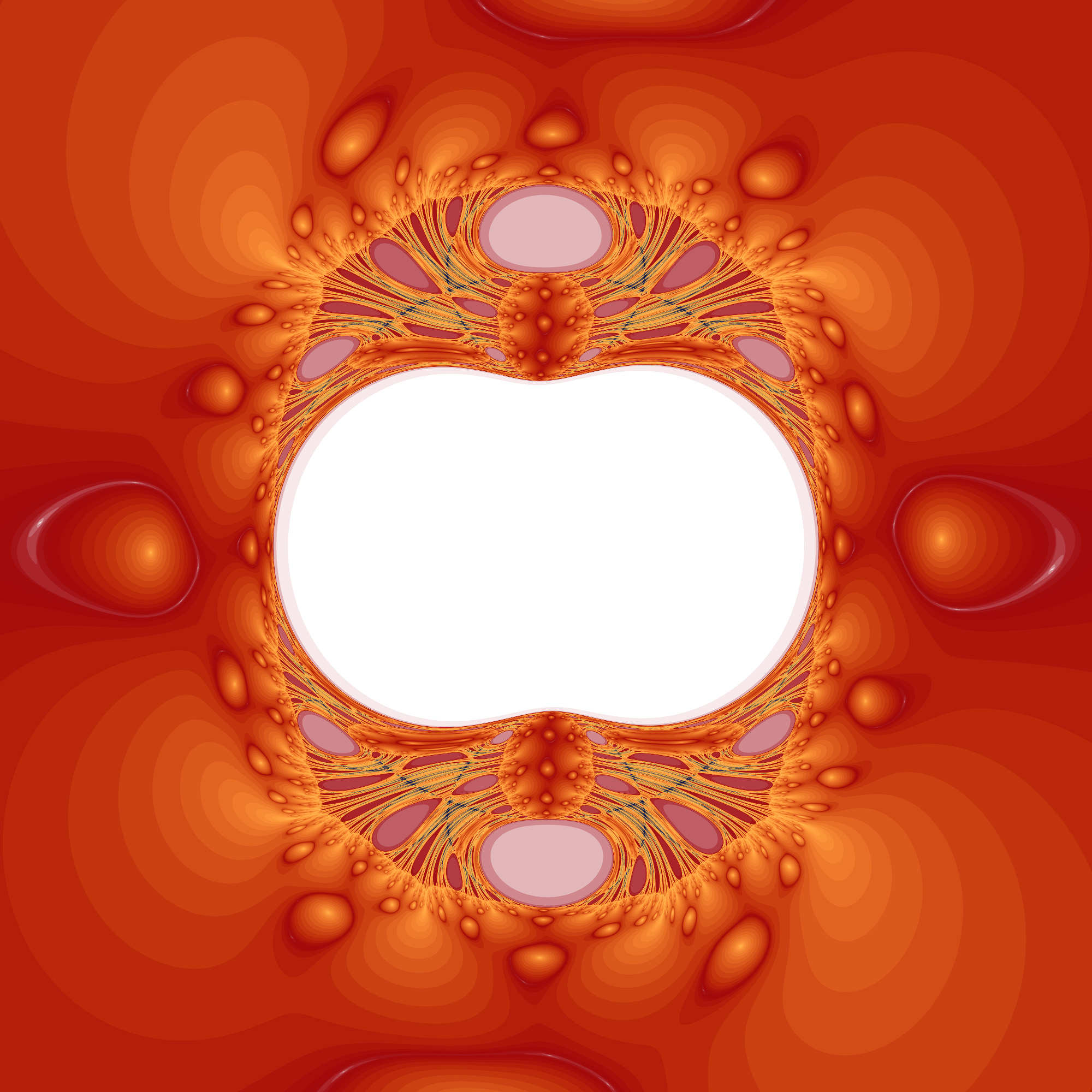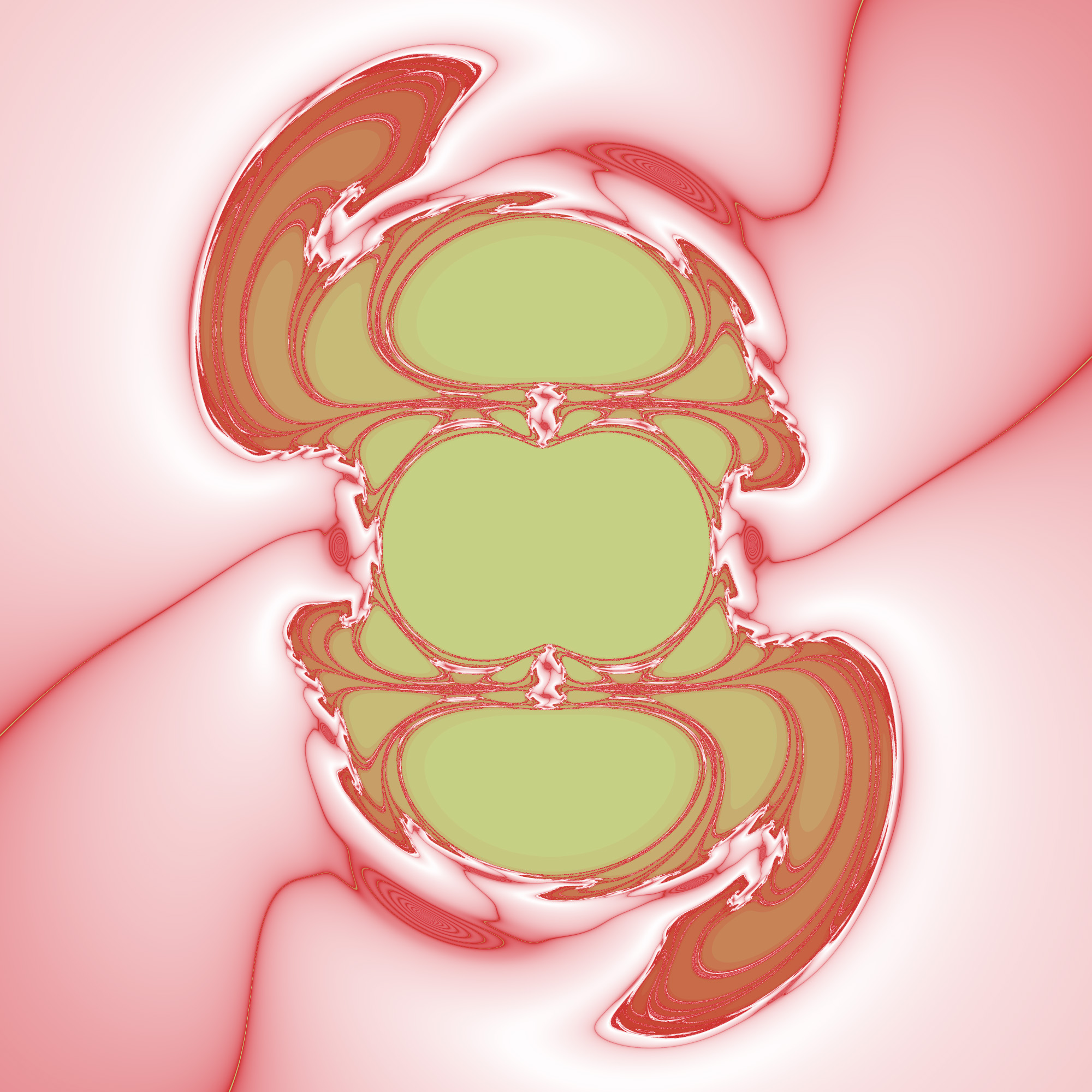# Insideout Dragon

Attributed to Roger Bagula

Graphics By Paul Bourke
July 2003

This fractal is calculated in a similar way to the Mandelbrot, that is, a series is calculated and coloured according to how quickly it escapes to infinity. The main difference is that the series in this case is somewhat more complicated. For a coordinate (x0,y0) one forms a complex number z0 = 1 / (x0 + i y0), this becomes the first term of the series.

The series is given by
zn+1 = zn2 + f(|zn|) + i g(|zn|)
where
f(r) = r (1 + 2 r + r2) (r2 - 1) / (1 + r3)2
and
g(r) = r (1 - 2 r + r2) (r2 - 1) / (1 + r3)2

and where |z| denotes the modulus of the complex number z.Variations
Change denominator from (1 + r3)2 to (1 + r + r7)